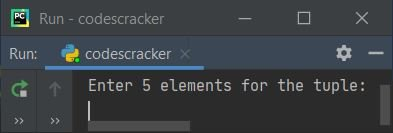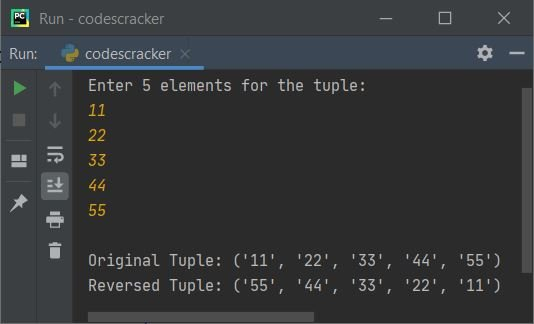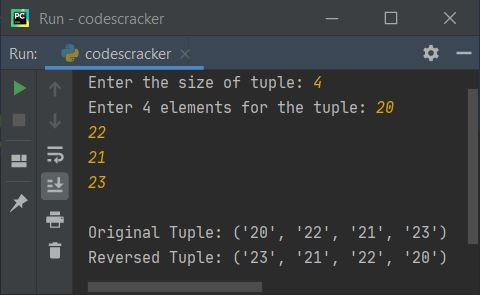# Python Program to Reverse a Tuple

This article is created to cover some programs in Python that find and prints reverse of a tuple entered by user at run-time. Here are the list of programs covered here:

• Reverse a Tuple
• Reverse a tuple of n items
• Reverse a Tuple by initializing Items one by one

## Reverse a Tuple

The question is, write a Python program to reverse a tuple. The items of tuple must be received by user. The answer to this question is the program given below:

```mylist = list()
print("Enter 5 elements for the tuple: ")
for i in range(5):
mylist.append(input())

mytuple = tuple(mylist)

revtuple = mytuple[::-1]

print("\nOriginal Tuple:", mytuple)
print("Reversed Tuple:", revtuple)```

Here is its initial output:Now provide the input say 11, 22, 33, 44, 55 as five elements for the tuple and press `ENTER` key to reverse the tuple like show in the snapshot given below:In above program, we've received items from user and stored in a list. After receiving items, we've created a tuple with exactly same elements stored in list (entered by user). Then further using [::-1], we've reversed the tuple like shown in the program and its sample run given above.

## Reverse a Tuple of n Items

This program allows user to define the size of tuple along with its items. Rest of the things are almost similar to previous program.

```mylist = list()
print("Enter the size of tuple: ", end="")
tot = int(input())
print("Enter", tot, "elements for the tuple: ", end="")
for i in range(tot):
mylist.append(input())

mytuple = tuple(mylist)

revtuple = mytuple[::-1]

print("\nOriginal Tuple:", mytuple)
print("Reversed Tuple:", revtuple)```

Here is its sample run with user input, 4 as size, 20, 22, 21, 23 as four elements:### Reverse a Tuple by initializing Items one by one

You can also use another technique to reverse a tuple like shown in the program given below. This program initializes the items of original tuple to the new tuple, but in reverse order. In this way, the tuple gets reversed.

```mylist = list()
print("Enter the size of tuple: ", end="")
tot = int(input())
print("Enter", tot, "elements for the tuple: ", end="")
for i in range(tot):
mylist.append(input())

mytuple = tuple(mylist)
print("\nOriginal Tuple:", mytuple)

revtuple = ()
for i in reversed(mytuple):
revtuple = revtuple + (i, )
print("Reversed Tuple:", revtuple)```

This program produces exactly same output as of previous program after providing exactly same inputs as of previous program's sample run.

Python Online Test

« Previous Program Next Program »